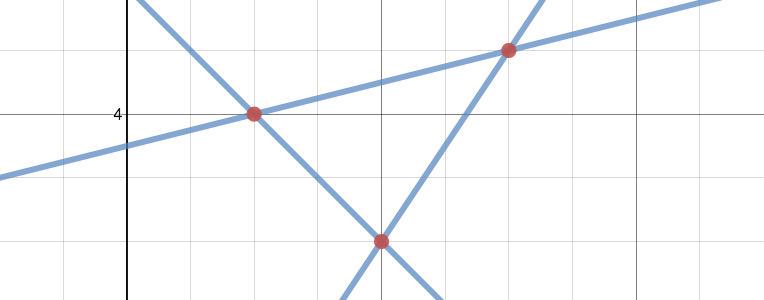# How Many Solutions?

I’ve been thinking about systems of linear equations for the past couple of days. Most of my focus has been on systems of two equations, but this morning I wrote a question about a system of three linear equations that might spark some interesting and revealing classroom conversations:And now I’m wondering…

• How many solutions does that system have? Drop your thoughts in the comments, would you?
• If you put this prompt in front of your students, I’d love to hear a recap of how that plays out.

1.Can we come up with a context for which the graph of the system above is a model? Perhaps water draining or filling in some type of containers. Certainly there is something more interesting. The solution then might depend on the question. When do all three containers have the same amount of liquid? At given moment in time which container has the most liquid?

2.It depends on what you want for a solution. If you want all three to be the same then there is no solution, but if you are just looking for 2 to be the same then there are 3 different solutions. It does all depend on what this system represents and what you are trying to find.

3.My vote is for no solutions. If we hold to definition that a solution satisfies an equation, and a solution to a system is any set of values which satisfy all equations in the system, then your graph shows no solutions.

4.5.I worked with some of my Algebra 1 8th graders on a systems of inequalities where there were 3 equations. It’s found here:

There needs to be some modification for my kids but it’s an awesome task. The issue boils down to “optimization” of the triply shaded area, which will be one of the points of intersection.

Not sure how to explain the the optimal point must be a point of intersection. Would love some help on this one…

6.I’m loving the how many solutions prompts. I use it in Algebra 2 to review all kinds of functions and compare. Helps with graphing, retention, etc.

7.This may be an interesting activity to ensure that students realize that the intersection of the lines represent the solution of a system. Students could be asked to write the three equations of the lines and list the three intersections. Then ask them to pair two equations for each intersection. A discussion of the three different systems and their solutions would help set the stage. I would then ask the students to answer the question about the solution to the system of the three equations. The hope would be that students would realize that if the solution was the point of intersection then all three lines would have to intersect for it to be a solution. Then the activity can move to asking the student to change one of the lines so that all three lines would intersect. For a real-world application consider some equilibrium context. Although the current equations may be “simple” for a truly real-world situation. It may be interesting to then move a system of inequalities – especially if the equations had a real-world context.

8.Post
Author

@peacewise: This is one of the things I love about the Internet: Here I am tossing an interesting but half-baked idea out there, and you’ve taken it and run with it, sketching out what sounds like a fantastic sequence of questions/prompts. I especially love the “change one line to the system has a solution” challenge. Thanks for sharing your ideas! 🙂

9.Similar to pejorgensPaul, I wish for a context to provide meaning for the search for a solution. Then, like squarepi and Peacewise, I saw the triangular region as representing a feasible region of possible solutions typical of linear programming problems.

My students and I encountered something similar when we searched for a location for a meeting place or a distribution center that was equal distance from several people or stores. Pairing several points to “construct” perpendicular bisectors usually produced a region from which to choose a optimal location. Simplifying the search to just three locations (points), represented by the vertices of a triangle, produces a single intersection point for the perpendicular bisectors (as long as the original points are not collinear).

GeoGebra is a nice tool for creating a dynamic representation to investigate under what conditions the intersections of the perpendicular bisector lines will coincide.

10.Post
Author

@pejorgensPaul and @mathgrip: I think applying a context to this could definitely lead to some interesting discussions. Here, I have in mind the context-less scenario because I’d like to explore whether—when trying to identify solutions to systems—students will simply hunt for intersections, or if they’ll keep in mind that a solution to a system of n equations must satisfy *all* n equations.

I find the “solution region” questions interesting as well, but think I would save that for our exploration of systems of linear inequalities (whereas I would use this question in my linear systems unit).

All that to say, I think both of your suggestions would certainly make for interesting alternative explorations.

11.@mjfenton, @peacewise Thank you for posing this question. I too am so excited to watch a lesson grow before my eyes as each person added a new insight. And now I am off to work up an activity borrowing ideas liberally from you both. Thanks for sharing!